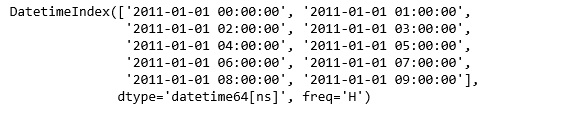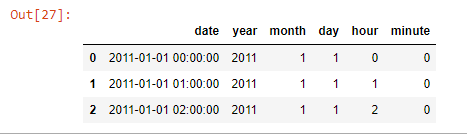# Python | Pandas Working with Dates and Times

• Last Updated : 15 Oct, 2018

Pandas has proven very successful as a tool for working with time series data, especially in the financial data analysis space. Using the NumPy datetime64 and timedelta64 dtypes, we have consolidated a large number of features from other Python libraries like scikits.timeseries as well as created a tremendous amount of new functionality for manipulating time series data.

Example #1: Create a dates dataframe

```import pandas as pd

# Create dates dataframe with frequency
data = pd.date_range('1/1/2011', periods = 10, freq ='H')

data
```

Output:Example #2: Create range of dates and show basic features

```# Create date and time with dataframe
data = pd.date_range('1/1/2011', periods = 10, freq ='H')

x = datetime.now()
x.month, x.year

```

Output:

`(9, 2018)`

Example #3: Break data and time into seperate features

```# Create date and time with dataframe
rng = pd.DataFrame()
rng['date'] = pd.date_range('1/1/2011', periods = 72, freq ='H')

# Print the dates in dd-mm-yy format
rng[:5]

# Create features for year, month, day, hour, and minute
rng['year'] = rng['date'].dt.year
rng['month'] = rng['date'].dt.month
rng['day'] = rng['date'].dt.day
rng['hour'] = rng['date'].dt.hour
rng['minute'] = rng['date'].dt.minute

# Print the dates divided into features

```

Output:Date Time Methods:

FunctionDescription
Timestamp()This constructor method is used with a variety of inputs (strings, date objects, date objects) to create some Timestamp objects
to_datetime()Method is used to convert various input arguements to datetime
date_range()Method is used to generate a DatetimeIndex of Timestamp objects. This constructor method includes 3 critical parameters (start, end, and periods)
date_range(start, periods)This approach creates a set number of dates beginning from a specific point
date_range(end, periods)This approach creates a set number of dates, proceeding backwards from a specified date point
DateOffsetThis object is used to add hours, days, weeks, months, and years to a DatetimeIndex
dt accessorIt allows us to access specific datetime properties on a Series of Timestamp object
loc[], .iloc[], and .ix[]Methods are used for extracting rows from a DataFrame with a DatetimeIndex
truncate()Method is used for slicing operations on objects with a DatetimeIndex. It includes two parameters — before and after — to specify the start and end of our date range

More on Pandas

My Personal Notes arrow_drop_up